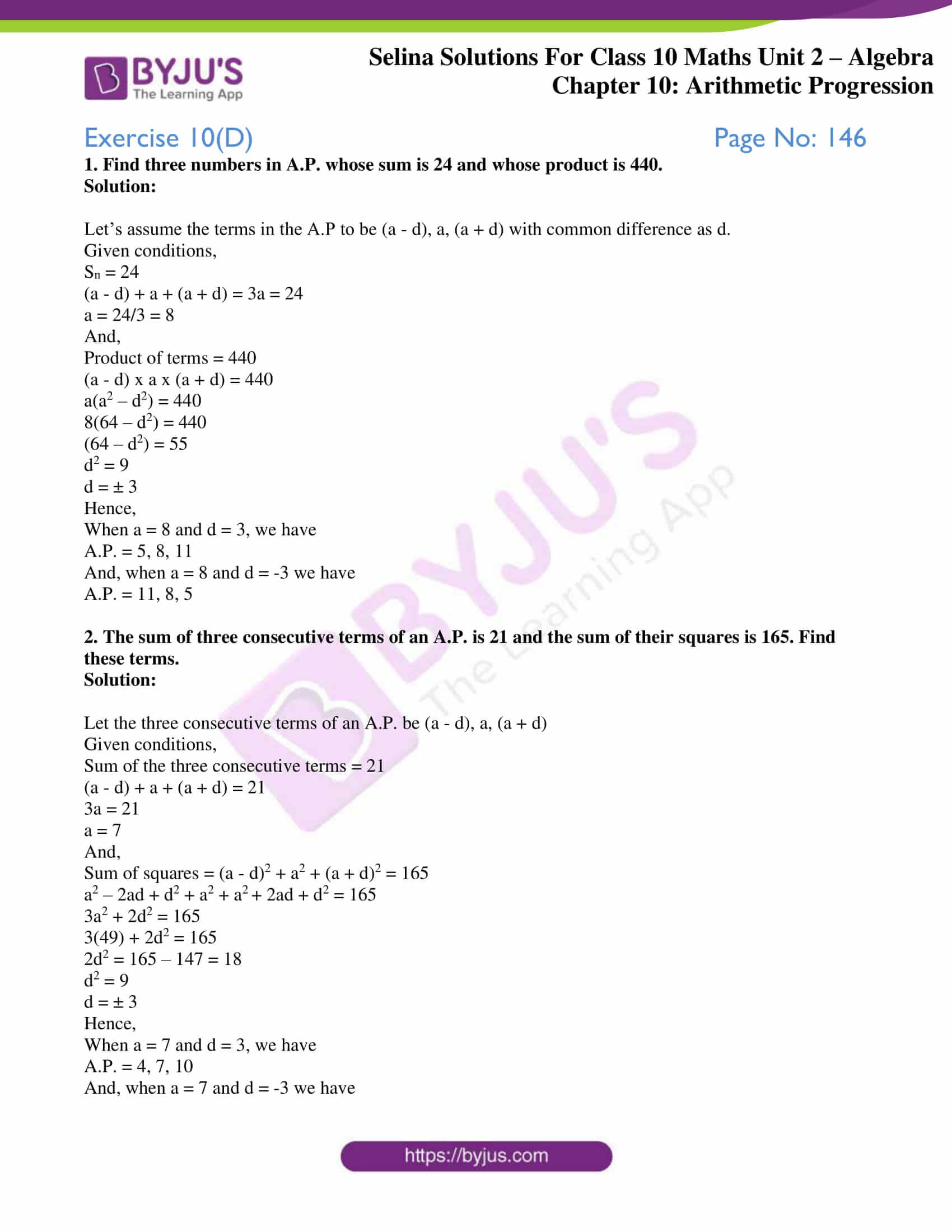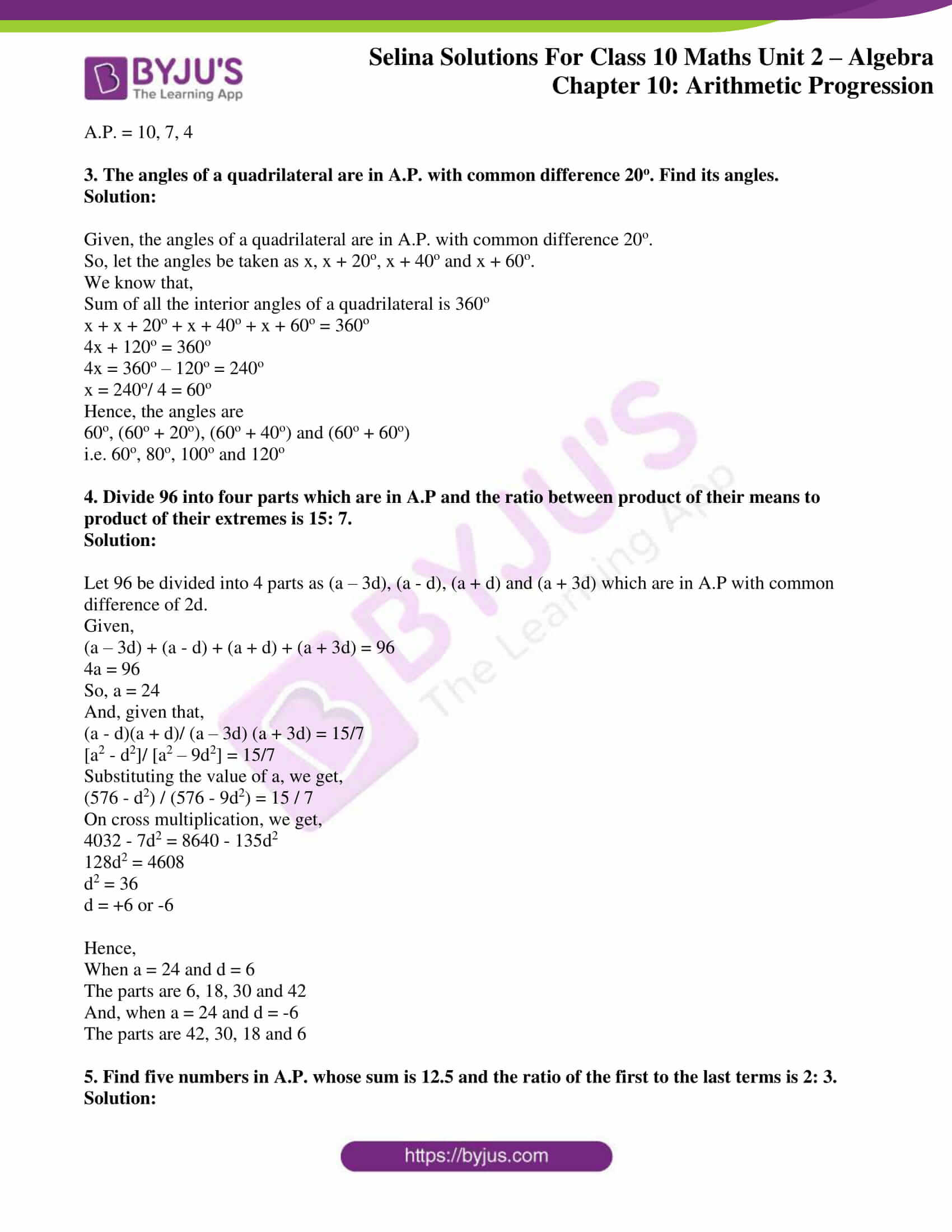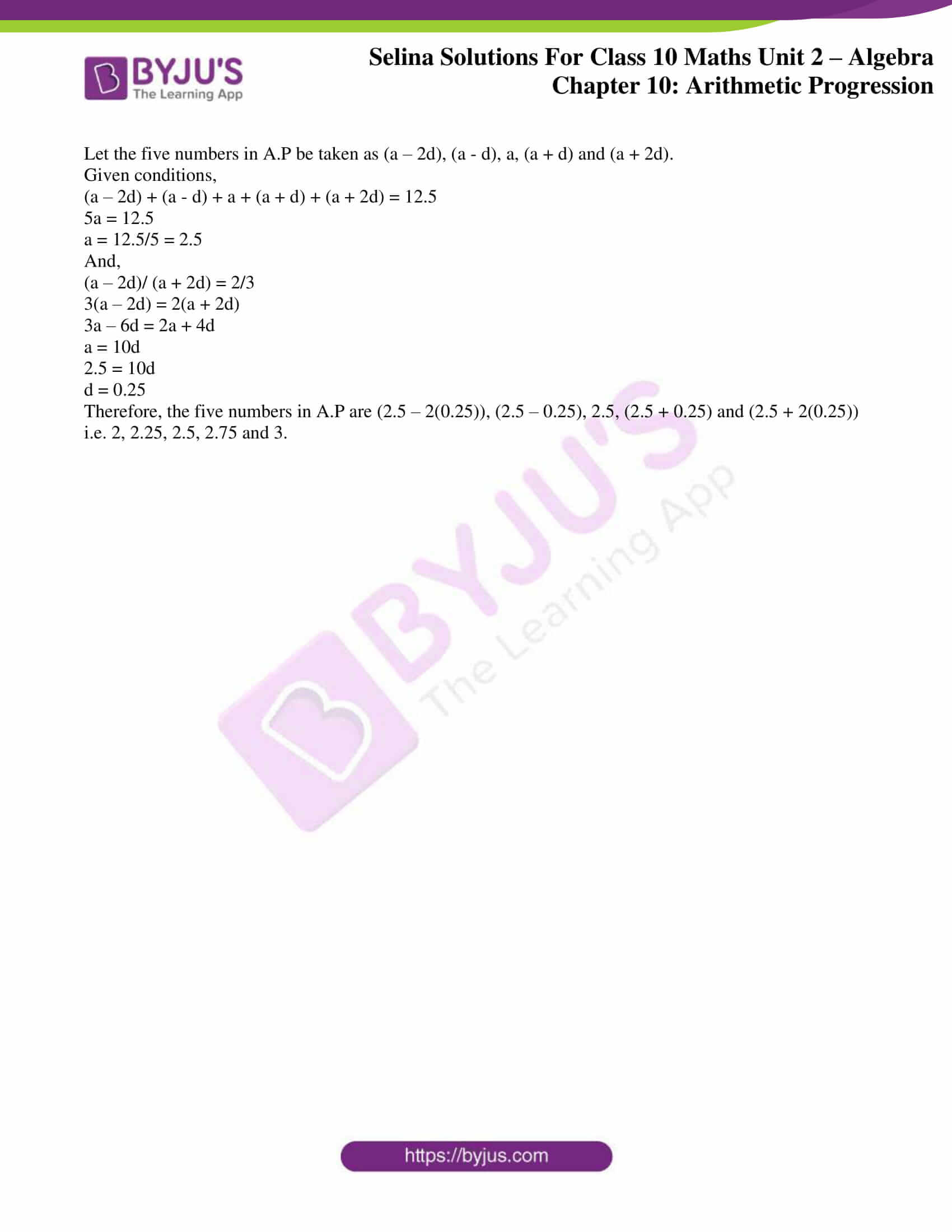# Selina Solutions Concise Maths Class 10 Chapter 10 Arithmetic Progression Exercise 10(D)

This exercise majorly deals with finding three or more terms in A.P, arithmetic mean and properties of an A.P. If students are facing difficulties in solving such problems then the Selina Solutions for Class 10 Maths is the right resource to overcome such difficulties. These solutions are developed by subject matter experts who have vast experience in the academic field. The solutions to this exercise are available in the Selina Solutions Concise Maths for Class 10 Chapter 10 Arithmetic Progression Exercise 10(D) PDF, from the links given below.

## Selina Solutions Concise Maths Class 10 Chapter 10 Arithmetic Progression Exercise 10(D) Download PDF### Access Selina Solutions Concise Maths Class 10 Chapter 10 Arithmetic Progression Exercise 10(D)

#### Exercise 10(D) Page No: 146

1. Find three numbers in A.P. whose sum is 24 and whose product is 440.

Solution:

Let’s assume the terms in the A.P to be (a – d), a, (a + d) with common difference as d.

Given conditions,

Sn = 24

(a – d) + a + (a + d) = 3a = 24

a = 24/3 = 8

And,

Product of terms = 440

(a – d) x a x (a + d) = 440

a(a2 – d2) = 440

8(64 – d2) = 440

(64 – d2) = 55

d2 = 9

d = ± 3

Hence,

When a = 8 and d = 3, we have

A.P. = 5, 8, 11

And, when a = 8 and d = -3 we have

A.P. = 11, 8, 5

2. The sum of three consecutive terms of an A.P. is 21 and the sum of their squares is 165. Find these terms.

Solution:

Let the three consecutive terms of an A.P. be (a – d), a, (a + d)

Given conditions,

Sum of the three consecutive terms = 21

(a – d) + a + (a + d) = 21

3a = 21

a = 7

And,

Sum of squares = (a – d)2 + a2 + (a + d)2 = 165

a2 – 2ad + d2 + a2 + a2 + 2ad + d2 = 165

3a2 + 2d2 = 165

3(49) + 2d2 = 165

2d2 = 165 – 147 = 18

d2 = 9

d = ± 3

Hence,

When a = 7 and d = 3, we have

A.P. = 4, 7, 10

And, when a = 7 and d = -3 we have

A.P. = 10, 7, 4

3. The angles of a quadrilateral are in A.P. with common difference 20o. Find its angles.

Solution:

Given, the angles of a quadrilateral are in A.P. with common difference 20o.

So, let the angles be taken as x, x + 20o, x + 40o and x + 60o.

We know that,

Sum of all the interior angles of a quadrilateral is 360o

x + x + 20o + x + 40o + x + 60o = 360o

4x + 120o = 360o

4x = 360o – 120o = 240o

x = 240o/ 4 = 60o

Hence, the angles are

60o, (60o + 20o), (60o + 40o) and (60o + 60o)

i.e. 60o, 80o, 100o and 120o

4. Divide 96 into four parts which are in A.P and the ratio between product of their means to product of their extremes is 15: 7.

Solution:

Let 96 be divided into 4 parts as (a – 3d), (a – d), (a + d) and (a + 3d) which are in A.P with common difference of 2d.

Given,

(a – 3d) + (a – d) + (a + d) + (a + 3d) = 96

4a = 96

So, a = 24

And, given that,

(a – d)(a + d)/ (a – 3d) (a + 3d) = 15/7

[a2 – d2]/ [a2 – 9d2] = 15/7

Substituting the value of a, we get,

(576 – d2) / (576 – 9d2) = 15 / 7

On cross multiplication, we get,

4032 – 7d2 = 8640 – 135d2

128d2 = 4608

d2 = 36

d = +6 or -6

Hence,

When a = 24 and d = 6

The parts are 6, 18, 30 and 42

And, when a = 24 and d = -6

The parts are 42, 30, 18 and 6

5. Find five numbers in A.P. whose sum is 12.5 and the ratio of the first to the last terms is 2: 3.

Solution:

Let the five numbers in A.P be taken as (a – 2d), (a – d), a, (a + d) and (a + 2d).

Given conditions,

(a – 2d) + (a – d) + a + (a + d) + (a + 2d) = 12.5

5a = 12.5

a = 12.5/5 = 2.5

And,

(a – 2d)/ (a + 2d) = 2/3

3(a – 2d) = 2(a + 2d)

3a – 6d = 2a + 4d

a = 10d

2.5 = 10d

d = 0.25

Therefore, the five numbers in A.P are (2.5 – 2(0.25)), (2.5 – 0.25), 2.5, (2.5 + 0.25) and (2.5 + 2(0.25))

i.e. 2, 2.25, 2.5, 2.75 and 3.

### Access other exercises of Selina Solutions Concise Maths Class 10 Chapter 10 Arithmetic Progression

Exercise 10(A) Solutions

Exercise 10(B) Solutions

Exercise 10(C) Solutions

Exercise 10(E) Solutions

Exercise 10(F) Solutions